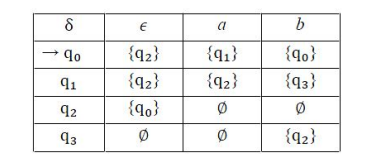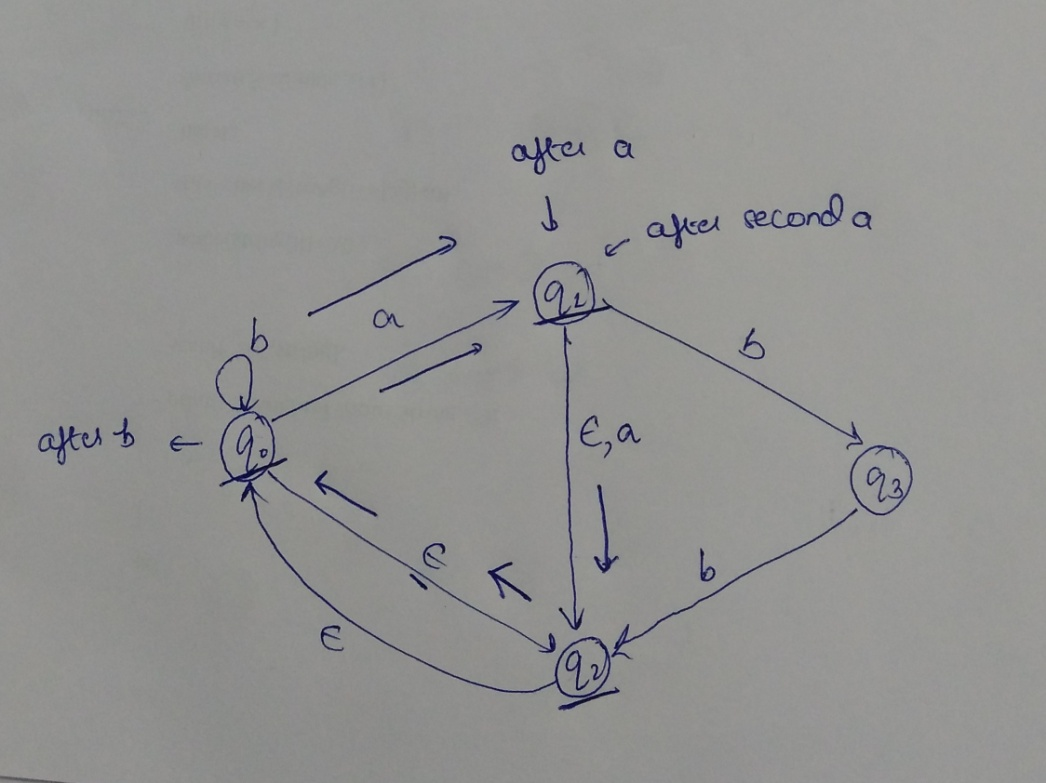# GATE | GATE-CS-2017 (Set 2) | Question 39

Let δ denote the transition function and α denoted the extended transition function of the ε-NFA whose transition table is given below:Then, α (q2,aba) is
(A) Ø
(B) {q1, q2, q3}
(C) {q0, q1, q2}
(D) {q0, q2, q3}

Explanation: Extended transition function describes what happens when we start in any state and follow any sequence of inputs.
Since this is an epsilon NFA so we have to consider the epsilon moves also and see which states we can reach after the input string is over.
The starting state is q2, from q2 transition with input a is dead so we have to look for epsilon transition.
With epsilon transition we reach to q0, at q0 we have a transition with input symbol a so we reach to state q1.
From q1 we can take transition with symbol b and reach state q3 but from q3 we have no further transition with symbol a as input so we have to take another transition from state q1 that is the epsilon transition which goes to state q2.
From q2 we reach to state q0 and read input b and then read input a and reach state q1.
So q1 is one of the state of extended transition function.
From q1 we can reach q2 with epsilon as input (mans with no input) and from q2 we can reach q0 with epsilon move so state q2 and q0 are also part of extended transition function.
So state q1, q2, q0This solution is contributed by Parul Sharma.

Quiz of this Question

My Personal Notes arrow_drop_up

Article Tags :

Be the First to upvote.

Please write to us at contribute@geeksforgeeks.org to report any issue with the above content.